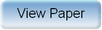Ninth International Geostatistics Congress, Oslo, Norway
June 11 – 15, 2012Session: Theory 3 Abstract No.: O-070 Title: Conditional simulation of a positive random vectorsubject to max-linear constraints. A geometric perspective. Author(s): C. Lantuéjoul, MinesParisTech (FR)F. MAISONNEUVE, Mines-ParisTech (FR)J.N. BACRO, Université de Montpellier (FR)L. BEL, AgroParisTech (FR) Abstract: Let \$X = ( X_1,X_2,...,X_p )\$ be a random vector with positive, independent and continuous components. The problem addressed in this presentation is the conditional simulation of \$X\$ subject to the fact that its components satisfy a set of \$n\$ max-linear constraints \$\max_j a_{ij} X_j = b_i\$ for \$i=1, \ldots, n\$. The coefficients \$a_{ij}\$ are nonnegative and the \$b_i\$'s are strictly positive. This problem is encountered in simulation studies of object-based processes and max-stable processes. It has been investigated by Wang and Stoev (2010, arXiv:1005.0312v1) using a purely algebraic approach. Our approach is more geometric and provides a number of complementary and sometimes unexpected results. It turns out that the set of vectors \$b=(b_1,...,b_n)\$ that make the \$n\$ constraints mutually compatible for a given matrix \$(a_ij)\$ has a very peculiar geometry. A consequence is that the set of conditional simulations does not vary continuously as a function of \$b\$. Suppose that the constraints are mutually compatible. Then it can be shown that each conditional simulation \$x=(x_1,...,x_p)\$ is necessarily located in the union of a number of faces of a certain polytope. An explicit expression of the conditional p.d.f. \$f(x)\$ is established that corroborates the results by Wang and Stoev. In particular \$f(x)=0\$ unless \$x\$ belongs to one of the faces of maximal dimension among the family of allowed faces. A critical problem is therefore the efficient identification of all allowed faces of maximal dimension, which is currently undergone using operational research techniques.Produced by X-CD Technologies Inc.